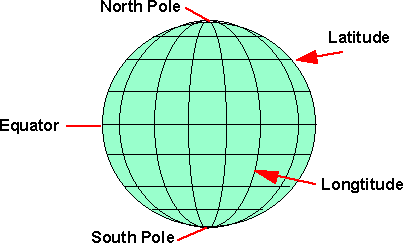# 3D Theory - Referencing

This is about how we measure the position on the surface of the earth, or possibly another planet.If we start by making an assumption that the earth is a perfect sphere, in this case we can use Spherical Polar Coordinates, as defined on this page. We can define a position on the earth using two angles:

• Latitude - an angle which is +90 degrees at the north pole, 0 degrees at the equator and -90 degrees at the south pole.
• Longitude - an angle about the line between the north and south poles, with 0 degrees at the Greenwich meridian.

We can assign an arbitrary x,y,z coordinate system in the local frame of the earth:so from this diagram we can see that:

z = r sin(latitude)

and if we are on the Greenwich meridian then:

x = r cos(latitude)

but if we are not on the Greenwich meridian then this has to be modified depending on the latitude, so,

x = r cos(latitude) cos (longitude)

the y can be calculated from:

r2 = x2 + y2 + z2

therefore y = r *sqrt(1 - sin(latitude) - cos(latitude) cos (longitude))

where:

r = radius of the earth (approx. 6378135 metres)

## Ellipsoid

The earth is not quite spherical, it is slightly flattened at the poles relative to the equator, this shape is known as an ellipsoid. This is a closer approximation, but not perfect, so many ellipsoids have been defined for different regions of the world.## Earth ellipsoids supported by x3d

 x3d Code Ellipsoid Name a - Equatorial Radius (metres) f - Flattening AA Airy 1830 6377563.396 1/299.3249646 AM Modified Airy 6377340.189 1/299.3249646 AN Australian National 6378160 1/298.25 BN Bessel 1841 (Namibia) 6377483.865 1/299.1528128 BR Bessel 1841 (Ethiopia Indonesia...) 6377397.155 1/299.1528128 CC Clarke 1866 6378206.4 1/294.9786982 CD Clarke 1880 6378249.145 1/293.465 EA Everest (India 1830) 6377276.345 1/300.8017 EB Everest (Sabah & Sarawak) 6377298.556 1/300.8017 EC Everest (India 1956) 6377301.243 1/300.8017 ED Everest (W. Malaysia 1969) 6377295.664 1/300.8017 EE Everest (W. Malaysia & Singapore 1948) 6377304.063 1/300.8017 EF Everest (Pakistan) 6377309.613 1/300.8017 FA Modified Fischer 1960 6378155 1/298.3 HE Helmert 1906 6378200 1/298.3 HO Hough 1960 6378270 1/297 ID Indonesian 1974 6378160 1/298.247 IN International 1924 6378388 1/297 KA Krassovsky 1940 6378245 1/298.3 RF Geodetic Reference System 1980 (GRS 80) 6378137 1/298.257222101 SA South American 1969 6378160 1/298.25 WD WGS 72 6378135 1/298.26 WE WGS 84 6378137 1/298.257223563

We can assign an arbitrary x,y,z coordinate system in the local frame of the earth:so from this diagram we can see that:

z = a * (1 -f) *sin(latitude)

and if we are on the Greenwich meridian then:

x = a cos(latitude)

but if we are not on the Greenwich meridian then this has to be modified depending on the latitude, so,

x = a cos(latitude) cos (longitude)

the y can be calculated from:

r2 = x2 + y2 + z2

therefore y = a *sqrt(1 - (1 -f) *sin(latitude) - cos(latitude) cos (longitude))

where:

• a = radius (on semimajor axis) of the earth
• f = flattening = (a - b) / a